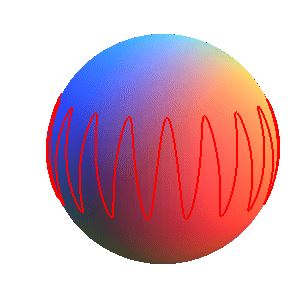# Spherical Sinusoid

Hello Grasshopper forum,

I am trying to map a sine curve onto a sphere using trigonometry and spherical coordinates but am struggling. Like this image:I have managed to get a sine curve to be mapped using cylindrical coordinates (definition attached):

Does anyone have a solution?

Much appreciated,

Cylindrical Sinusoid.gh (7.8 KB)

Thanks dowazura, but that method uses the pull curve component to map the sine curve onto the sphere, I was trying to find a solution which uses only an equation (as a challenge). The screenshot I sent is misleading, you gave me the result, just not in the way I am looking for.

hp = wave height parameter between -1 and 1
n = number of waves
rotational angle = 0 to 2 * pi

elevation angle = n * rotation angle
rflat = radius * cos(elevation angle)
x = rflat * cos(theta)
y = rflat * sin(theta)
z = radius * sin(elevation angle)

Or use spherical coordinates (radius, rotational angle, elevation angle)

1 Like

This is perfect, thanks!